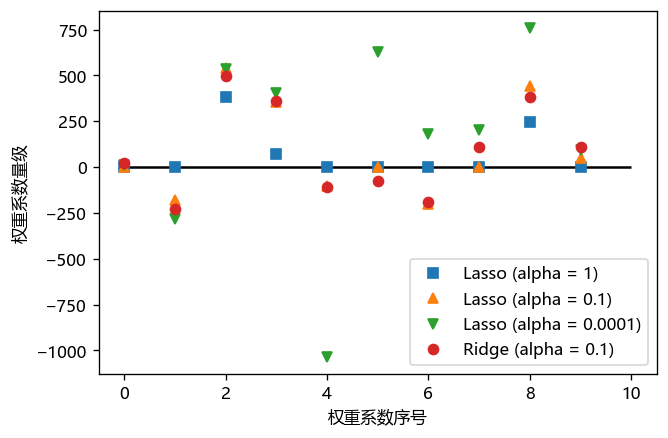# 广义线性模型¶

## 1. 线性模型的基本概念¶

### 1.1 线性模型的数学表达¶

$\hat{y} = w*x + w*x + ... + w[p]*x[p] + b$

• $x,x,...x[p]$ 是数据集中的特征变量;
• 参数 $p$ 表示每个样本都有 $p$ 个特征；
• $w$ 和 $b$ 是模型的参数；
• $\hat{y}$ 是模型对给定数据的预测结果， $\hat{y}$ 读作：y hat，一般来说 hat 表示估计值。。

$\hat{y} = w*x + b$

In :
import numpy as np
import matplotlib.pyplot as plt

# 配置参数使 matplotlib绘图工具可以显示中文
plt.rcParams['font.sans-serif'] = [u'Microsoft YaHei']

# 设置自变量 x：令x为-5到5之间，元素数量为100的等差数列
x = np.linspace(-5, 5, 100)

# 按照方程的数学表达式，定义直线方程
y = 0.5*x + 3

# 设置绘图内容的基本参数
plt.plot(x, y, c = "blue")
# 设置图的题目
plt.title("直线 Straight Line")
# 激活绘图功能，在坐标轴上显示直线
plt.show()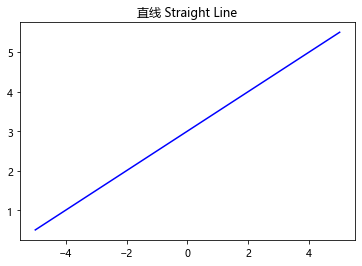### 1.2 线性模型的可视化¶

#### 1.2.1 基于两个数据点的模型拟合和可视化¶

• 拟合样本及可视化

In :
# 导入线性回归模型
from sklearn.linear_model import LinearRegression
import numpy as np
import matplotlib.pyplot as plt

# 配置参数使 matplotlib绘图工具可以显示中文
plt.rcParams['font.sans-serif'] = [u'Microsoft YaHei']

# 输入两个点的坐标，分别保存在矩阵 x 和 y 中
X = [, ]
y = [3, 5]

# 使用线性模型拟合给定的样本点
lr = LinearRegression().fit(X, y)

# 画出点和基于点生成的直线
z = np.linspace(0, 5, 20) # 生成 0到5之间，元素个数为20的等差数列
z = z.reshape(-1, 1) # 将矩阵转换为 n 行，1列的矩阵

plt.scatter(X, y, s = 80)
# 可视化预测结果，其中：
# 横坐标： 变量 z;
# 纵坐标： 基于 变量 z 和 线性模型 lr 生成的预测值。
# 第三个参数c：线的颜色
plt.plot(z, lr.predict(z), c = "k")

# 设定图的显示信息并显示图片
plt.title("直线 Straight Line")
plt.show()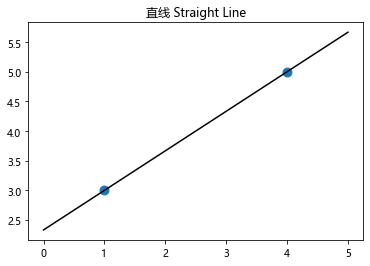In :
z = np.linspace(0, 5, 20)
z

Out:
array([0.        , 0.26315789, 0.52631579, 0.78947368, 1.05263158,
1.31578947, 1.57894737, 1.84210526, 2.10526316, 2.36842105,
2.63157895, 2.89473684, 3.15789474, 3.42105263, 3.68421053,
3.94736842, 4.21052632, 4.47368421, 4.73684211, 5.        ])

X = [[a1, b1, c1], [a2, b2, c2]] y = [y1, y2]
• 生成拟合后的直线方程
In :
print("直线方程为：\n")
print("y = {:.3f}".format(lr.coef_), "x", "+ {:.3f}".format(lr.intercept_))

直线方程为：

y = 0.571 x + 2.143


#### 1.2.2 基于三个数据点的模型拟合和可视化¶

• 拟合样本及可视化
In :
# 导入线性回归模型
from sklearn.linear_model import LinearRegression
import numpy as np
import matplotlib.pyplot as plt

# 配置参数使 matplotlib绘图工具可以显示中文
plt.rcParams['font.sans-serif'] = [u'Microsoft YaHei']

# 输入三个点的坐标，分别保存在矩阵 x 和 y 中
X = [, , ]
y = [3, 5, 3]

# 使用线性模型来拟合这3个样本点
lr = LinearRegression().fit(X, y)

# 画出点和基于点生成的直线
z = np.linspace(0, 5, 20) # 生成 0到5之间，元素个数为20的等差数列
z = z.reshape(-1, 1) # 将矩阵转换为 n 行，1列的矩阵

plt.scatter(X, y, s = 80)
# 可视化预测结果，其中：
# 横坐标： 变量 z;
# 纵坐标： 基于 变量 z 和 线性模型 lr 生成的预测值。
# 第三个参数c：线的颜色
plt.plot(z, lr.predict(z), c = "k")

# 设定图的显示信息并显示图片
plt.title("直线 Straight Line")
plt.show()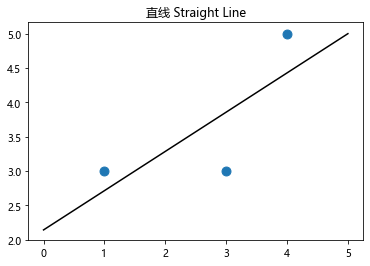• 生成拟合后的直线方程
In :
print("直线方程为：\n")
print("y = {:.3f}".format(lr.coef_), "x", "+ {:.3f}".format(lr.intercept_))

直线方程为：

y = 0.571 x + 2.143


#### 1.2.3 基于多样本的模型拟合和可视化¶

• 拟合样本及可视化
In :
# 导入线性回归模型等多个库
import numpy as np
import matplotlib.pyplot as plt
from sklearn.linear_model import LinearRegression
from sklearn.datasets import make_regression

# 配置参数使 matplotlib绘图工具可以显示中文
plt.rcParams['font.sans-serif'] = [u'Microsoft YaHei']

# 使用make_regression()函数生成数据集
X, y = make_regression(n_samples = 50, n_features = 1, n_informative = 1,
noise = 50, random_state = 1)

# 使用线性模型来拟合这3个样本点
lr = LinearRegression().fit(X, y)

# 画出点和基于点生成的直线
z = np.linspace(-3, 3, 200) # 生成 -3到3之间，元素个数为200的等差数列
z = z.reshape(-1, 1) # 将矩阵转换为 n 行，1列的矩阵

plt.figure(dpi=150)
plt.scatter(X, y, c = "b", s = 40) # s为散点的尺度
# 可视化预测结果，其中：
# 横坐标： 变量 z;
# 纵坐标： 基于 变量 z 和 线性模型 lr 生成的预测值。
# 第三个参数c：线的颜色
plt.plot(z, lr.predict(z), c = "k")

# 设定图的显示信息并显示图片
plt.title("线性回归")
plt.show()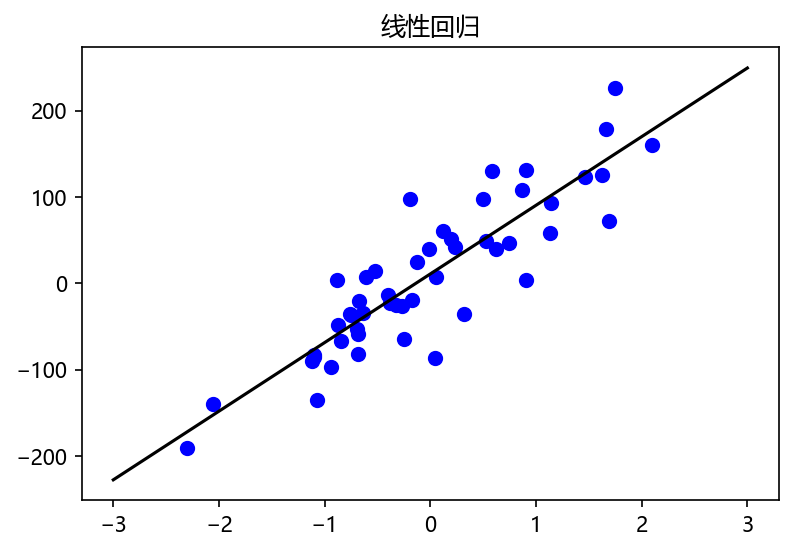【知识点】[reshape(-1, 1)](functions/reshape.ipynb)

• 生成拟合后的直线方程
In :
print("直线方程为：\n")
w = lr.coef_
b = lr.intercept_
print("y = {:.3f}".format(w), "x", "+ {:.3f}".format(b))
print("其中，权重系数为: {0:.3f}, 截距为: {1:.3f}。".format(w, b))

直线方程为：

y = 79.525 x + 10.922



### 1.3 线性模型的优缺点¶

• 优点
• 建模速度快，不需要复杂的计算，特别是在大数据量下依然具有较快的运算速度
• 可以根据系数给出每个变量的理解和解释
• 缺点
• 不能很好拟合非线性数据，因此需要先判断变量间是否具有线性关系

1. 线性回归能够模拟的数据远不止线性关系，并且回归中的“线性"指的是系数的线性，通过特征的非线性变换及广义线性模型的推广，输出和特征之间可以是高度非线性的；
2. 线性模型的易解释性让它在物理学、经济学、商学等领域具有不可替代的地位。

## 2. 最基本的线性模型——线性回归（Linear Regression）¶

### 2.1 线性回归模型的基本原理¶

1. 使用make_regression()函数生成一个样本数为100，特征数为2的的数据集
2. 使用train_test_split()函数对数据集进行划分
3. 使用线性回归模型计算权重 w 和 截距 b 的值，并给出回归模型的方程
4. 性能分析
In :
# 0.导入数据集生成工具和拆分工具
from sklearn.linear_model import LinearRegression
from sklearn.model_selection import train_test_split
from sklearn.datasets import make_regression

# 1. 使用make_regression()函数生成一个样本数为1000，特征数为2的的数据集
X, y = make_regression(n_samples = 1000, n_features = 2, n_informative = 2, random_state = 38, noise = 0)

# 2. 使用train_test_split()函数对数据集进行划分
X_train, X_test, y_train, y_test = train_test_split(X, y, random_state = 8, test_size = 0.7)

# 3. 使用线性回归模型计算权重 *w* 和 截距 *b* 的值，并给出回归模型的方程
lr = LinearRegression().fit(X_train, y_train)
w = lr.coef_
b = lr.intercept_

# 显示回归模型的方程及参数
print("lr.coef_: {}".format(w))
print("lr.intercept_: {}".format(b))
print("回归方程为：")
print("y = {0:.3f}*x1 + {1:.3f}*x2 + {2}".format(w, w, b))

lr.coef_: [ 0.47026977 72.59456432]
lr.intercept_: 0.0

y = 0.470*x1 + 72.595*x2 + 0.0


In :
# 4. 性能分析
score_train = lr.score(X_train, y_train)
score_test = lr.score(X_test, y_test)
print("训练集得分: {:.3f}".format(score_train))
print("测试集得分: {:.3f}".format(score_test))

训练集得分: 1.000



Surprise!

In :
# 0.导入数据集生成工具和拆分工具
from sklearn.linear_model import LinearRegression
from sklearn.model_selection import train_test_split

# 1. 使用make_regression()函数生成一个样本数为1000，特征数为2的的数据集
X, y = make_regression(n_samples = 1000, n_features = 2, n_informative = 2, random_state = 38, noise = 30)

# 2. 使用train_test_split()函数对数据集进行划分
X_train, X_test, y_train, y_test = train_test_split(X, y, random_state = 8, test_size = 0.7)

# 3. 使用线性回归模型计算权重 *w* 和 截距 *b* 的值，并给出回归模型的方程
lr = LinearRegression().fit(X_train, y_train)

# 4. 性能分析
score_train = lr.score(X_train, y_train)
score_test = lr.score(X_test, y_test)
print("训练集得分: {:.3f}".format(score_train))
print("测试集得分: {:.3f}".format(score_test))

训练集得分: 0.851



### 2.2 基于线性回归模型的糖尿病数据集分析¶

In :
# 0.导入数据集生成工具和拆分工具
from sklearn.linear_model import LinearRegression
from sklearn.model_selection import train_test_split

# 1. 载入糖尿病情数据集

# 2. 使用train_test_split()函数对数据集进行划分
X_train, X_test, y_train, y_test = train_test_split(X, y, random_state = 8)

# 3. 使用线性回归模型计算权重 *w* 和 截距 *b* 的值，并给出回归模型的方程
lr = LinearRegression().fit(X_train, y_train)

# 4. 性能分析
score_train = lr.score(X_train, y_train)
score_test = lr.score(X_test, y_test)
print("训练集得分: {:.3f}".format(score_train))
print("测试集得分: {:.3f}".format(score_test))

训练集得分: 0.530



## 3. 使用L2正则化的线性模型——岭回归（Ridge Regression）¶

### 3.2 基于岭回归的预测¶

#### 3.2.1 岭回归在"糖尿病情"数据集上的应用¶

In :
# 0.导入数据集生成工具和拆分工具
from sklearn.linear_model import Ridge
from sklearn.model_selection import train_test_split

# 1. 载入糖尿病情数据集

# 2. 使用train_test_split()函数对数据集进行划分
X_train, X_test, y_train, y_test = train_test_split(X, y, random_state = 8)

# 3. 使用线性回归模型计算权重 *w* 和 截距 *b* 的值，并给出回归模型的方程
ridge = Ridge().fit(X_train, y_train)

# 4. 性能分析
score_train = ridge.score(X_train, y_train)
score_test = ridge.score(X_test, y_test)
print("训练集得分: {:.3f}".format(score_train))
print("测试集得分: {:.3f}".format(score_test))

训练集得分: 0.433



1. 无论是训练集，还是测试集的预测结果，岭回归的结果都要差于线性回归。原因可能是样本数量较少
2. 训练集和测试集之间的误差非常小。这说明，岭回归所用的L2约束有效地降低了过拟合的问题。换句话说岭回归比线性模型具有更强的泛化能力。

**【知识点】**模型泛化: 泛化能力（generalization ability）是指机器学习算法对新鲜样本的适应能力。 学习的目的是学到隐含在数据背后的规律，对具有同一规律的学习集以外的数据，经过训练的网络也能给出合适的输出，该能力称为泛化能力。

#### 3.2.2 岭回归在"波士顿房价"数据集上的应用¶

In :
# 1. 载入波士顿房价数据集
from sklearn.linear_model import Ridge
from sklearn.model_selection import train_test_split

# 或许数据集中的数据和标签

# 2. 使用train_test_split()函数对数据集进行划分
X_train, X_test, y_train, y_test = train_test_split(X, y, random_state = 8)

# 3. 使用线性回归模型计算权重 *w* 和 截距 *b* 的值，并给出回归模型的方程
Ridge = Ridge().fit(X_train, y_train)

# 4. 性能分析
score_train = Ridge.score(X_train, y_train)
score_test = Ridge.score(X_test, y_test)
print("训练集得分: {:.3f}".format(score_train))
print("测试集得分: {:.3f}".format(score_test))

训练集得分: 0.743



#### 3.2.3 岭回归的参数调节¶

【知识点】机器学习的超参数没有绝对性，通常需要根据数据集的不同进行调节。超参数的选择是一个非常经验性的工作，也是一个非常困难的工作，需要程序员在长期的工作中总结和归纳。当然，当前已经有不少的算法用于实现超参数的选择和搜索。

In :
# 0.导入数据集生成工具和拆分工具
from sklearn.linear_model import Ridge
from sklearn.model_selection import train_test_split

# 1. 载入糖尿病情数据集

# 2. 使用train_test_split()函数对数据集进行划分
X_train, X_test, y_train, y_test = train_test_split(X, y, random_state = 8)

# 3. 使用线性回归模型计算权重 *w* 和 截距 *b* 的值，并给出回归模型的方程
ridge = Ridge(alpha = 10).fit(X_train, y_train)

# 4. 性能分析
score_train = ridge.score(X_train, y_train)
score_test = ridge.score(X_test, y_test)
print("训练集得分: {:.3f}".format(score_train))
print("测试集得分: {:.3f}".format(score_test))

训练集得分: 0.151



In :
# 3. 使用线性回归模型计算权重 *w* 和 截距 *b* 的值，并给出回归模型的方程
ridge = Ridge(alpha = 0.1).fit(X_train, y_train)

# 4. 性能分析
score_train = ridge.score(X_train, y_train)
score_test = ridge.score(X_test, y_test)
print("训练集得分: {:.3f}".format(score_train))
print("测试集得分: {:.3f}".format(score_test))

训练集得分: 0.522



In :
# 3. 使用线性回归模型计算权重 *w* 和 截距 *b* 的值，并给出回归模型的方程
ridge = Ridge(alpha = 0).fit(X_train, y_train)

# 4. 性能分析
score_train = ridge.score(X_train, y_train)
score_test = ridge.score(X_test, y_test)
print("训练集得分: {:.3f}".format(score_train))
print("测试集得分: {:.3f}".format(score_test))

训练集得分: 0.530



#### 3.2.4 更详细的性能分析¶

1. alpha对coef_属性的影响

In :
# 0.导入数据集生成工具和拆分工具
from sklearn.linear_model import Ridge
from sklearn.linear_model import LinearRegression
from sklearn.model_selection import train_test_split
import matplotlib.pyplot as plt

# 配置参数使 matplotlib绘图工具可以显示中文
plt.rcParams['font.sans-serif'] = [u'Microsoft YaHei']

# 1. 载入糖尿病情数据集

# 2. 使用train_test_split()函数对数据集进行划分
X_train, X_test, y_train, y_test = train_test_split(X, y, random_state = 8)

# 3. 使用线性回归模型计算权重 *w* 和 截距 *b* 的值，并给出回归模型的方程
lr = LinearRegression().fit(X_train, y_train)
ridge0 = Ridge(alpha = 0).fit(X_train, y_train)
ridge01 = Ridge(alpha = 0.1).fit(X_train, y_train)
ridge1 = Ridge(alpha = 1).fit(X_train, y_train)
ridge10 = Ridge(alpha = 10).fit(X_train, y_train)

# 4. 将超参数 alpha 对 参数coef_ 的影响进行可视化
# 分别绘制线性回归模型, alpha = [0, 0.1, 1, 10]时的图形
plt.figure(dpi=120) # 设置图形的尺度
plt.plot(lr.coef_, "s", label = "线性回归模型")
plt.plot(ridge0.coef_, "^", label = "岭回归模型(alpha=0)")
plt.plot(ridge01.coef_, "o", label = "岭回归模型(alpha=0.1)")
plt.plot(ridge1.coef_, "<", label = "岭回归模型(alpha=1)")
plt.plot(ridge10.coef_, "+", label = "岭回归模型(alpha=10)")

plt.xlabel("权重系数序号")
plt.ylabel("权重系数量级")
plt.hlines(0, 0, len(lr.coef_)) # 绘制当前坐标轴上的水平线
plt.legend(loc='best')

Out:
<matplotlib.legend.Legend at 0x26ad6341f08>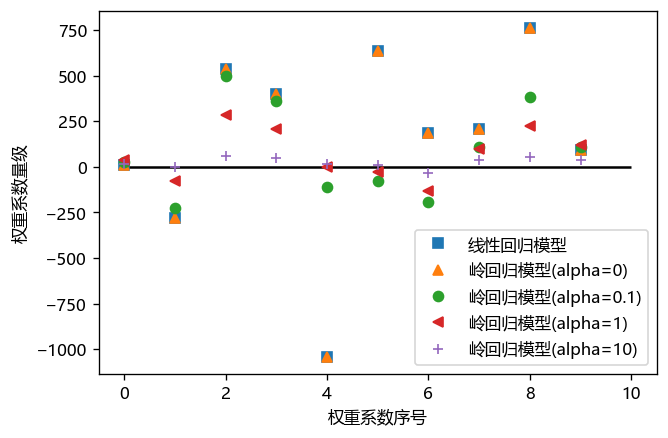1. 超参数 alpha = 10 (较大时)，特征变量的系数接近于0，L2正则化的约束较严
2. 超参数 alpha = 1 时，特征系数变大，L2正则化的约束被放松
3. 超参数 alpha = 0.1 时，特征系数继续增大，L2正则化的约束几乎失去作用
4. 超参数 alpha = 0 时，特征系数值和线性回归完全重合，L2正则化的约束也彻底失去作用

【函数说明】[plt.legend](functions/plt.legend.ipynb) [plt.plot](functions/plt.plot.ipynb)

1. 训练集大小对模型性能的影响
In :
# 0.导入数据集生成工具和拆分工具
import numpy as np
from sklearn.linear_model import Ridge
from sklearn.linear_model import LinearRegression
from sklearn.model_selection import learning_curve, KFold
import matplotlib.pyplot as plt

# 配置参数使 matplotlib绘图工具可以显示中文
plt.rcParams['font.sans-serif'] = [u'Microsoft YaHei']

# 1. 载入糖尿病情数据集

# 定义一个用于绘制学习曲线的函数
def plot_learning_curve(est, X, y):
# 将数据进行20次拆分来对模型进行评分
training_set_size, train_scores, test_scores = learning_curve(est, X, y, train_sizes = np.linspace(0.1, 1, 20),
cv = KFold(20, shuffle = True, random_state = 1))
estimator_name = est.__class__.__name__
line = plt.plot(training_set_size, train_scores.mean(axis = 1), '--', label = "训练集 " + estimator_name)

# plt.plot()参数解释：x轴，y轴，图标样式，图例（数组类型），色彩
plt.plot(training_set_size, test_scores.mean(axis = 1), '-',
label = "测试集 " + estimator_name, c = line.get_color())
plt.xlabel("训练集样本数")
plt.ylabel("模型评分")
plt.ylim(0, 1.1) # y轴坐标范围

plt.figure(dpi = 120) # 设置图形的尺度
plot_learning_curve(Ridge(alpha = 1), X, y)
plot_learning_curve(LinearRegression(), X, y)
plt.legend(loc = "best")

Out:
<matplotlib.legend.Legend at 0x26ad20f3948>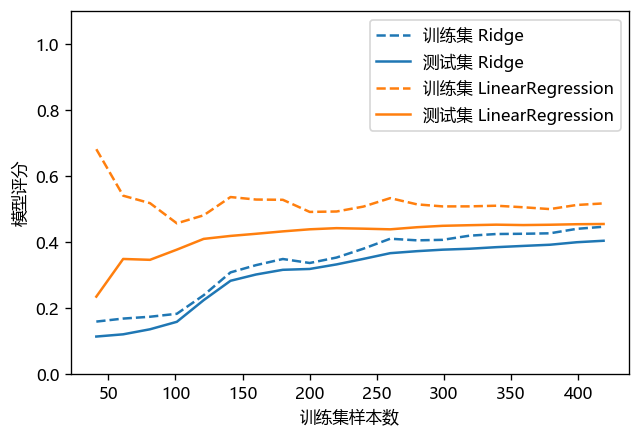1. 训练集评分始终高于测试集;
2. 岭回归的训练集和测试集之间的差距较小，基本没有过拟合问题，泛化性能较强;
3. 随着训练集样本数量的增加，两个模型测试集的性能也在提高
4. 随着训练集样本数量的增加，岭回归Ridge的性能区域稳定，且性能几乎和线性回归一致。说明数据量的增加使L2正则化的用处逐渐降低；
5. 随着训练集样本数量的增加，线性回归训练集的性能逐渐下降。说明随着数据量的增加，线性回归出现过拟合问题的几率逐渐下降，或者说越难学到训练集的知识。

=> **[重点知识]** 训练样本的增加(样本数量、样本类型)，(在一定程度上)是有助于提高系统性能

【函数说明】[learning_curve](functions/learning_curve.ipynb) ：绘制学习曲线

## 4. 使用L1正则化的线性模型——套索回归（Lasso Regression）¶

### 4.1 套索回归的原理¶

（此处暂时忽略这种算法的优势，有兴趣的同学可以Baidu一下，特别是Dropout算法）

### 4.2 基于套索回归的预测¶

#### 4.2.1 套索回归在"糖尿病"数据集上的应用¶

In :
# 0.导入数据集生成工具和拆分工具
from sklearn.linear_model import Lasso
from sklearn.model_selection import train_test_split
import numpy as np

# 1. 载入糖尿病情数据集

# 2. 使用train_test_split()函数对数据集进行划分
X_train, X_test, y_train, y_test = train_test_split(X, y, random_state = 8)

In :
# 3. 使用线性回归模型计算权重 *w* 和 截距 *b* 的值，并给出回归模型的方程
lasso = Lasso().fit(X_train, y_train)

# 4. 性能分析
score_train = lasso.score(X_train, y_train)
score_test = lasso.score(X_test, y_test)
print("训练集得分: {:.3f}".format(score_train))
print("测试集得分: {:.3f}".format(score_test))
print("样本的特征数量: {0}; 模型所使用特征的数量: {1}".format(X_train.shape, np.sum(lasso.coef_ != 0)))

训练集得分: 0.362



1. 实验结果非常糟糕，并且训练集和测试集的结果都很糟糕，这说明模型发生了欠拟合问题。即模型无法很好地学到训练数据的特征。"过拟合和欠拟合"的问题将在后续的课程中，以专题形式探讨。
2. 样本具有10个特征而套索模型仅仅使用了3个特征，因此对于该数据集来说，特征学习度是显然不足的

#### 4.2.2 套索回归的优化¶

In :
# 3. 使用线性回归模型计算权重 *w* 和 截距 *b* 的值，并给出回归模型的方程
lasso = Lasso(alpha = 0.1, max_iter = 100000).fit(X_train, y_train)

# 4. 性能分析
score_train = lasso.score(X_train, y_train)
score_test = lasso.score(X_test, y_test)
print("训练集得分: {:.3f}".format(score_train))
print("测试集得分: {:.3f}".format(score_test))
print("样本的特征数量: {0}; 模型所使用特征的数量: {1}".format(X_train.shape, np.sum(lasso.coef_ != 0)))

训练集得分: 0.519



1. 超参数alpha的降低，使欠拟合的问题基本消失了，但过拟合的问题逐渐显现
2. 由于欠拟合问题的解决，模型的性能有一定的提高。

In :
# 3. 使用线性回归模型计算权重 *w* 和 截距 *b* 的值，并给出回归模型的方程
lasso = Lasso(alpha = 0.0001, max_iter = 100000).fit(X_train, y_train)

# 4. 性能分析
score_train = lasso.score(X_train, y_train)
score_test = lasso.score(X_test, y_test)
print("训练集得分: {:.3f}".format(score_train))
print("测试集得分: {:.3f}".format(score_test))
print("样本的特征数量: {0}; 模型所使用特征的数量: {1}".format(X_train.shape, np.sum(lasso.coef_ != 0)))

训练集得分: 0.530



#### 4.2.3 套索回归和岭回归的比较¶

In :
# 0.导入数据集生成工具和拆分工具
import numpy as np
from sklearn.linear_model import Ridge
from sklearn.linear_model import Lasso
from sklearn.model_selection import train_test_split
import matplotlib.pyplot as plt

# 配置参数使 matplotlib绘图工具可以显示中文
plt.rcParams['font.sans-serif'] = [u'Microsoft YaHei']

# 1. 载入糖尿病情数据集

# 2. 使用train_test_split()函数对数据集进行划分
X_train, X_test, y_train, y_test = train_test_split(X, y, random_state = 8)

lasso1 = Lasso(alpha = 1, max_iter = 100000).fit(X_train, y_train)
lasso01 = Lasso(alpha = 0.1, max_iter = 100000).fit(X_train, y_train)
lasso00001 = Lasso(alpha = 0.0001, max_iter = 100000).fit(X_train, y_train)
ridge01 = Ridge(alpha = 0.1).fit(X_train, y_train)

plt.figure(dpi = 120)
plt.plot(lasso1.coef_, 's', label = "Lasso (alpha = 1)")
plt.plot(lasso01.coef_, '^', label = "Lasso (alpha = 0.1)")
plt.plot(lasso00001.coef_, 'v', label = "Lasso (alpha = 0.0001)")
plt.plot(ridge01.coef_, 'o', label = "Ridge (alpha = 0.1)")
plt.legend(loc = "best")
plt.xlabel("权重系数序号")
plt.ylabel("权重系数量级")
plt.hlines(0, 0, len(ridge01.coef_)) # 绘制当前坐标轴上的水平线

Out:
<matplotlib.collections.LineCollection at 0x26ad1fdda08>